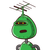# General form of linear equation in two variables x and y is​

General form of linear equation in two variables x and y is

### 1 thought on “<br /><br />General form of linear equation in two variables x and y is<br />​”

1.Step-by-step explanation:

linear equation is ax+by+c=0